# Stat-Ease Blog

## Randomization Done Right

posted by Shari Kraber on Sept. 8, 2022

Randomization is essential for success with planned experimentation (DOE) to protect factor effects against bias by lurking variables. For example, consider the 8-run, two-level factorial design shown in Table 1. It lays out the low (−) and high (+) coded levels of each factor in standard, not random, order. Notice that factor C changes level only once throughout the experiment—first being set at the low (minus) level for four runs, followed by the remaining four runs set at the high (plus) level. Now, let’s say that the humidity in the room increases throughout the day—affecting the measured response. Since the DOE runs are not randomized, the change in humidity biases the calculated effect of the non-randomized factor C. Therefore, the effect of factor C includes the humidity change – it is no longer purely due to the change from low to high. This will cause analysis problems!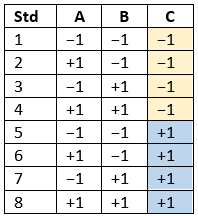Table 1: Standard order of 8-run design

Randomization itself presents some problems. For example, one possible random order is the classic standard layout, which, as you now know, does not protect against time-related effects. If this unlikely pattern, or other non-desirable patterns are seen, then you should re-randomize the runs to reduce the possibility of bias from lurking variables.

#### Randomizing center points or other replicates

Replicates, such as center points, are used to collect information on the pure error of the system. To optimize the validity of this information, center points should be spaced out over the experimental run order. Random order may inadvertently place replicates in sequential order. This requires manual intervention by the researcher to break up or separate the repeated runs so that each run is completed independently of the matching run.

In both Design-Expert® software and Stat-Ease 360 you can re-randomize by right-clicking on the Run column header and selecting Randomize, as shown in Figure 1. You can also simply edit the Run order and swap two runs by changing the run numbers manually. This is often the easiest method when you want to separate center points, for example.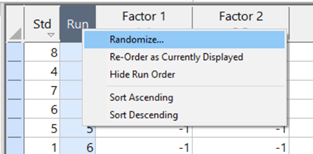Figure 1: Right-click to Randomize

#### When Randomization Doesn’t Work

While randomization is ideal statistically, sometimes it is cumbersome in practice. For instance, temperature can take a very long time to change, so completely randomizing the runs may cause the experiment to go way beyond the time budget. In this case, researchers look for ways to reduce the complete randomization of the design.

I want to highlight a common DOE mistake. An incorrect way to restrict the randomization is to use blocks. Blocking is a statistical technique that groups the experimental runs to eliminate a potential source of variation from the data analysis. A common blocking factor is “day”, setting the block groups to eliminate day-to-day variation. Although this is a form of restricting randomization, if you block on an experimental factor like temperature, then statistically the block (temperature) effect will be removed from the analysis. Any interaction effect with that block will also be removed. The removal of this key effect very likely destroys the entire analysis! Blocking is not a useful method for restricting the randomization of a factor that is being studied in the experiment. For more information on why you would block, see “Blocking: Mowing the Grass in Your Experimental Backyard”.

If factor changes need to be restricted (not fully randomized), then building a split-plot design is the best way to go. A split-plot design takes into account the hard-to-change versus easy-to-change factors in a restricted randomization test plan. Perfect! The associated analysis properly assesses the differences in variation between these two groups of factors and provides the correct effect evaluation. The statistical analysis is a bit more complex, but good DOE software will handle it easily. Split-plot designs are a more complex topic, but commonly used in today’s experimental practices. Learn more about split-plot designs in this YouTube video: Split Plot Pros and Cons – Dealing with a Hard-to-Change Factor.

#### Wrapping up

Randomization is essential for valid and unbiased factor effect calculations, which is central to effective design of experiments analysis. It is up to the experimenter to ensure that the randomization of the experimental runs meets the DOE goals. Manual intervention may be required to separate any replicated points, such as center points. If complete randomization is not possible from a practical standpoint, build a split-plot design that statistically accounts for those restrictions.

## Understanding Lack of Fit: When to Worry

posted by Shari Kraber on May 4, 2022

An analysis of variance (ANOVA) is often accompanied by a model-validation statistic called a lack of fit (LOF) test. A statistically significant LOF test often worries experimenters because it indicates that the model does not fit the data well. This article will provide experimenters a better understanding of this statistic and what could cause it to be significant.

The LOF formula is: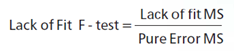where MS = Mean Square. The numerator (“Lack of fit”) in this equation is the variation between the actual measurements and the values predicted by the model. The denominator (“Pure Error”) is the variation among any replicates. The variation between the replicates should be an estimate of the normal process variation of the system. Significant lack of fit means that the variation of the design points about their predicted values is much larger than the variation of the replicates about their mean values. Either the model doesn't predict well, or the runs replicate so well that their variance is small, or some combination of the two.

Case 1: The model doesn’t predict well

On the left side of Figure 1, a linear model is fit to the given set of points. Since the variation between the actual data and the fitted model is very large, this is likely going to result in a significant LOF test. The linear model is not a good fit to this set of data. On the right side, a quadratic model is now fit to the points and is likely to result in a non-significant LOF test. One potential solution to a significant lack of fit test is to fit a higher-order model.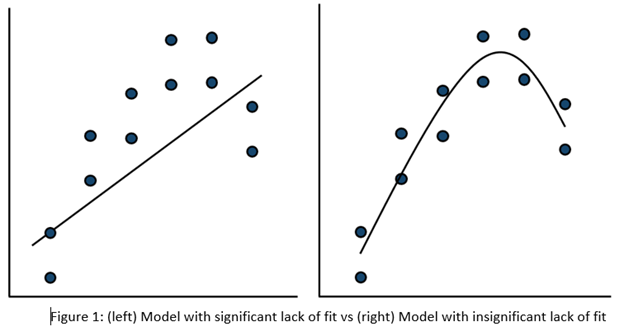Case 2: The replicates have unusually low variability

Figure 2 (left) is an illustration of a data set that had a statistically significant factorial model, including some center points with variation that is similar to the variation between other design points and their predictions. Figure 2 (right) is the same data set with the center points having extremely small variation. They are so close together that they overlap. Although the predicted factorial model fits the model points well (providing the significant model fit), the differences between the actual data points are substantially greater than the differences between the center points. This is what triggers the significant LOF statistic. The center points are fitting better than the model points. Does this significant LOF require us to declare the model unusable? That remains to be seen as discussed below.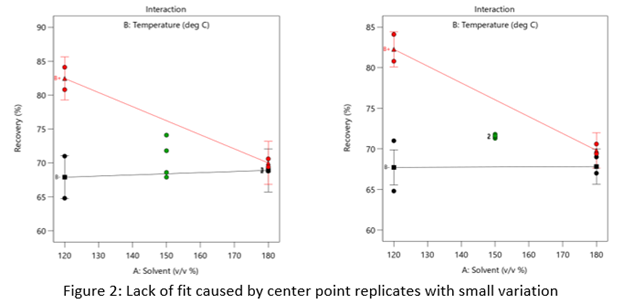When there is significant lack of fit, check how the replicates were run— were they independent process conditions run from scratch, or were they simply replicated measurements on a single setup of that condition? Replicates that come from independent setups of the process are likely to contain more of the natural process variation. Look at the response measurements from the replicates and ask yourself if this amount of variation is similar to what you would normally expect from the process. If the “replicates" were run more like repeated measurements, it is likely that the pure error has been underestimated (making the LOF denominator artificially small). In this case, the lack of fit statistic is no longer a valid test and decisions about using the model will have to be made based on other statistical criteria.

If the replicates have been run correctly, then the significant LOF indicates that perhaps the model is not fitting all the design points well. Consider transformations (check the Box Cox diagnostic plot). Check for outliers. It may be that a higher-order model would fit the data better. In that case, the design probably needs to be augmented with more runs to estimate the additional terms.

If nothing can be done to improve the fit of the model, it may be necessary to use the model as is and then rely on confirmation runs to validate the experimental results. In this case, be alert to the possibility that the model may not be a very good predictor of the process in specific areas of the design space.

## Improving Your Predictive Model via a Response Transformation

posted by Shari Kraber on Jan. 5, 2022

A good predictive model must exhibit overall significance and, ideally, insignificant lack of fit plus high adjusted and predicted R-squared values. Furthermore, to ensure statistical validity (e.g., normality, constant variance) the model’s residuals must pass a series of diagnostic tests (fortunately made easy by Stat-Ease software):

• Normal plot of residuals illustrates a relatively straight line. If you can cover the residuals with a fat pencil, no worries, but watch out for a pronounced S-shaped curve such as Figure 1 exhibits.
• Residuals-versus-predicted plot has points scattered randomly, i.e., demonstrating a constant variance from left to right. Beware of a “megaphone” shape as seen in Figure 2.
• Residuals-versus-run plot exhibiting no trends, shifts or outliers (points outside the red lines such as seen in Figure 3).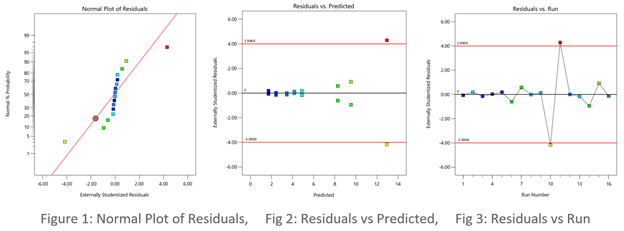When diagnostic plots of residuals do not pass the tests, the first thing you should consider for a remedy is a response transformation, e.g., rescaling the data via a natural log (again made easy by Stat-Ease software). Then re-fit the model and re-check the diagnostic plots. Often you will see improvements in both the statistics and the plots of residuals.

The Box-Cox plot (see Figure 4) makes the choice of transformation very simple. Based on the fitted model, this diagnostic displays a comparable measure of residuals against a range of power transformations, e.g., taking the inverse of all your responses (lambda -1), or squaring them all (lambda 2). Obviously, the lower the residuals the better. However, only go for a transformation if your current responses at the power of 1 (the blue line), fall outside the red-lined confidence interval, such as Figure 4 display. Then, rather than going to the exact-optimal power (green line), select one that will be simpler (and easier to explain)--the log transformation in this case (conveniently recommended by Stat-Ease software).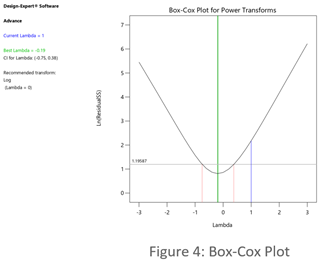See the improvement made by the log transformation in the diagnostics (Figures 5, 6 and 7). All good!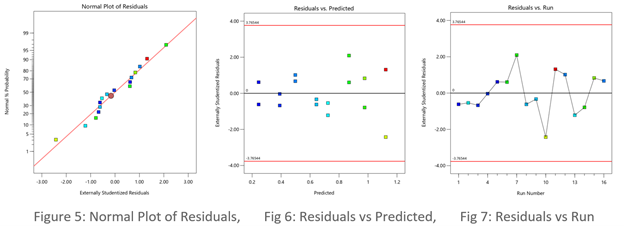In conclusion, before pressing ahead with any model (or abandoning it), always check the residual diagnostics. If you see any strange patterns, consider a response transformation, particularly if advised to do so by the Box-Cox plot. Then confirm the diagnostics after re-fitting the model.

For more details on diagnostics and transformations see How to Use Graphs to Diagnose and Deal with Bad Experimental Data.

Good luck with your modeling!

~ Shari Kraber, shari@statease.com

## R-Squared Mysteries Solved

posted by Shari Kraber on Nov. 9, 2021

Design of experiments (DOE) and the resulting data analysis yields a prediction equation plus a variety of summary statistics. A set of R-squared values are commonly used to determine the goodness of model fit. In this blog, I peel back the raw versus adjusted versus predicted R-squared and explain how each can be interpreted, along with the relationships between them. The calculations of these values can be easily found online, so I won’t spend time on that, focusing instead on practical interpretations and tips.

Raw R-squared measures the fraction of variation explained by the fitted predictive model. This is a good statistic for comparing models that all have the same number of terms (like comparing models consisting of A+B versus A+C). The downfall of this statistic is that it can be artificially increased simply by adding more terms to the model, even ones that are not statistically significant. For example, notice in Table 1 from an optimization experiment how R-squared increases as the model steps up in order from linear to two-factor interaction (2FI), quadratic and, finally, cubic (disregarding it being aliased).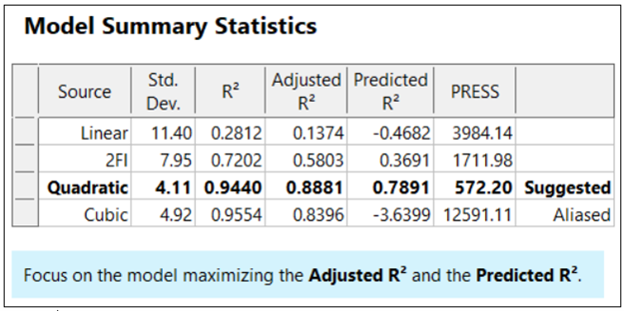The “adjusted” R-squared statistic corrects this ‘inflation’ by penalizing terms that do not add statistical value. Thus, the adjusted R-squared statistic generally levels off (at 0.8881 in this case) and then begins to decrease at some point as seen in Table 1 for the cubic model (0.8396). The adjusted R-squared value cannot be inflated by including too many model terms. Therefore, you should report this measure of model fit, not the raw R-squared.

The “predicted” R-squared is most rigorous for assessing model fit, so much so that it often starts off negative at the linear order, as it does for the example in Table 1 (-0.4682). As you can see, this statistic improves greatly as significant terms are added to the model, and quickly decreases once non-significant terms are added, e.g., going negative again at cubic. If predicted R-squared goes negative, the model becomes worse than nothing, that is, simply taking the average of the data (a “mean” model)—that is not good!

Figures 1 illustrates how the predicted R-squared peaks at the quadratic model for the example. Once a model emerges at the highest adjusted and/or predicted R-squared, consider taking out any insignificant terms—best done with the aid of a computerized reduction algorithm. This often produces a big increase in the predicted R-squared.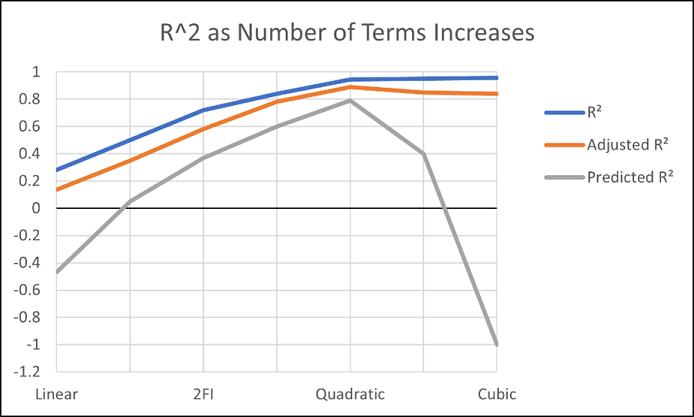Conclusion

The goal of modeling data is to correctly identify the terms that explain the relationship between the factors and the response. Use the adjusted R-squared and predicted R-squared values to evaluate how well the model is working, not the raw R-squared.

PS: You’ve likely been reading this expecting to find recommended adjusted and predicted R-squared values. I will not be providing this. Higher values indicate that more variation in the data or in predictions is explained by the model. How you use the model dictates the threshold that is acceptable to you. If the DOE goal is screening, low values can be acceptable. Remember that low R-squared values do not invalidate significant p-values. In other words, if you discover factors that have strong effects on the response, that is positive information, even if the model doesn’t predict well. A low predicted R-squared means that there is more unexplained variation in the system, and you have more work to do!

## Why it pays to be skeptical of three-factor-interaction effects

posted by Mark Anderson on Sept. 29, 2021

Quite often, when providing statistical help for Stat-Ease software users, our consulting team sees an over-selection of effects from two-level factorial experiments. Generally, the line gets crossed when picking three-factor interactions (3FI), as I documented in the lead article for the June 2007 Stat-Teaser. In this case, the experimenter picked all the estimable effects when only one main effect (factor B) really stood out on the Pareto plot. Check it out!

In my experience, the true 3FIs emerge only when one of the variables is categorical with a very strong contrast. For example, early in my career as an R&D chemical engineer with General Mills, I developed a continuous process for hydrogenating a vegetable oil. By cranking up the pressure and temperature and using an expensive, noble-metal catalyst (palladium on a fixed bed of carbon), this new approach increased the throughput tremendously over the old batch process, which deployed powered nickel to facilitate the reaction. When setting up my factorial experiment, our engineering team knew better than to make the type of reactor one of the inputs, because being so different, this would generate many complications of time-temperature interactions differing from on process to the other. In cases like this, you are far better off doing separate optimizations and then seeing which process wins out in the end. (Unfortunately for me, I lost this battle due to the color bodies in the oil poisoning my costly catalyst.)

A response must really behave radically to require a 3FI for modeling as illustrated hypothetically in Figures 1 versus 2 for two factors—catalyst level (B) and temperature (D)—as a function of a third variable (E)—the atmosphere in the reactor.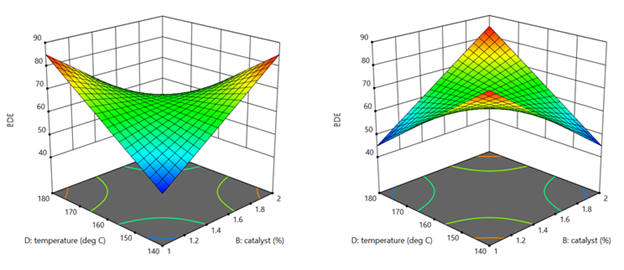Figures 1 & 2: 3FI (BDE) surface with atmosphere of nitrogen vs air (Factor E at low & high levels)

These surfaces ‘flip-flop’ completely like a bird in flight. Although factor E being categorical does lead to a strong possibility of complex behavior from this experiment, the dramatic shift caused by it changing from one level to the other would be highly unusual by my reckoning.

It turns out that there is a middle ground with factorial models that obviates the need for third-order terms: Multiple two-factor interactions (2FIs) that share common factors. The actual predictive model, derived from a case study we present in our Modern DOE for Process Optimization workshop, is:

Yield = 63.38 + 9.88*B + 5.25*D − 3.00*E + 6.75*BD − 5.38*DE

Notice that this equation features two 2FIs, BD and DE, that share a common factor (D). This causes the dynamic behavior shown in Figures 3 and 4 without the need for 3FI terms.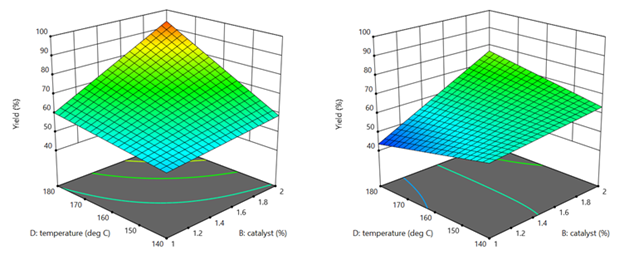Figure 3 & 4: 2FI surface (BD) for atmosphere of nitrogen vs air (Factor E at low & high levels)

This simpler model sufficed to see that it would be best to blanket the batch reactor with nitrogen, that is, do not leave the hatch open to the air—a happy ending.

Conclusion

If it seems from graphical or other methods of effect selection that 3FI(s) should be included in your factorial model, be on guard for:

• Over-selection of effects (my first case)
• The need for a transformation (such as log): Be sure to check the Box-Cox plot (always!).
• Outlier(s) in your response (look over the diagnostic plots, especially the residual versus run).
• A combination of these and other issues—ask stathelp@statease.com for guidance if you use Stat-Ease software (send in the file, please).

I never say “never”, so if you really do find a 3FI, get back to me directly.

-Mark (mark@statease.com)